## Box2D C++ tutorials - Ray casting

Last edited: July 14 2013

Chinese version -> 中文

### Ray casting

Ray casting is often used to find out what objects are in a certain part of the world. A ray is just a straight line, and we can use a function provided by Box2D to check if the line crosses a fixture. We can also find out what the normal is at the point the line hits the fixture.

Here is the function I'm talking about, which returns true if the ray hits the fixture. Notice it's a member of the b2Fixture class, which means we'll first need to have one of those to cast a ray against.
 ```1 ``` `  bool b2Fixture::RayCast(b2RayCastOutput* output, const b2RayCastInput& input);`
Now what are these input and output parameters. Well, straight from the source code here is what a b2RayCastInput contains:
 ```1 2 3 4 5 6 ``` ```  // Ray-cast input data. The ray extends from p1 to p1 + maxFraction * (p2 - p1).   struct b2RayCastInput   {       b2Vec2 p1, p2;       float32 maxFraction;   };```
The points p1 and p2 are used to define a direction for the ray, and the maxFraction specifies how far along the ray should be checked for an intersection. The following image may make this clearer. A maxFraction of 1 simply means the segment from p1 to p2, which in this case would not intersect the shape, but a maxFraction of 2 would.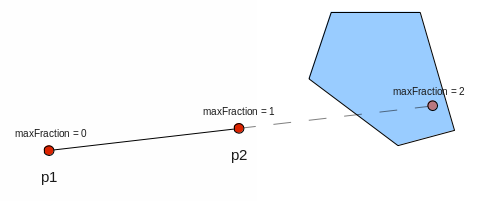And here is what a b2RayCastOutput contains:
 ```1 2 3 4 5 6 7 ``` ```  // Ray-cast output data. The ray hits at p1 + fraction * (p2 - p1), where p1 and p2   // come from b2RayCastInput.   struct b2RayCastOutput   {       b2Vec2 normal;       float32 fraction;   };```
If the ray does intersect the shape, b2Fixture::RayCast will return true and we can look in the output struct to find the actual fraction of the intersect point, and the normal of the fixture 'surface' at that point: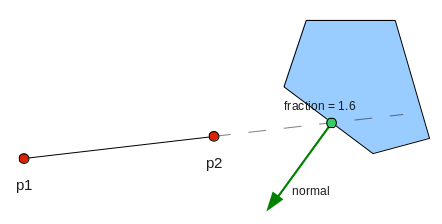### Example

To try out this very handy function, let's set up a scene with a fenced area and some shapes floating inside in a zero-gravity environment. By now you should be getting used to this one, so we'll make the walls as edges instead of boxes just to spice things up.
 ```1 2 3 4 5 6 7 8 9 10 11 12 13 14 15 16 17 18 19 20 21 22 23 24 25 26 27 28 29 30 31 32 33 34 35 36 37 38 39 40 41 42 43 44 45 46 47 ``` ```    FooTest() {         //a static body       b2BodyDef myBodyDef;       myBodyDef.type = b2_staticBody;       myBodyDef.position.Set(0, 0);       b2Body* staticBody = m_world->CreateBody(&myBodyDef);         //shape definition       b2PolygonShape polygonShape;           //fixture definition       b2FixtureDef myFixtureDef;       myFixtureDef.shape = &polygonShape;             //add four walls to the static body       b2Vec2 bl(-20, 0);       b2Vec2 br( 20, 0);       b2Vec2 tl(-20,40);       b2Vec2 tr( 20,40);       polygonShape.SetAsEdge( bl, br ); //ground       staticBody->CreateFixture(&myFixtureDef);       polygonShape.SetAsEdge( tl, tr);//ceiling       staticBody->CreateFixture(&myFixtureDef);       polygonShape.SetAsEdge( bl, tl );//left wall       staticBody->CreateFixture(&myFixtureDef);       polygonShape.SetAsEdge( br, tr );//right wall       staticBody->CreateFixture(&myFixtureDef);         myBodyDef.type = b2_dynamicBody;       myBodyDef.position.Set(0,20);       polygonShape.SetAsBox(2,2);       myFixtureDef.density = 1;       for (int i = 0; i < 5; i++)           m_world->CreateBody(&myBodyDef)->CreateFixture(&myFixtureDef);         //circles       b2CircleShape circleShape;       circleShape.m_radius = 2;       myFixtureDef.shape = &circleShape;       for (int i = 0; i < 5; i++)           m_world->CreateBody(&myBodyDef)->CreateFixture(&myFixtureDef);         //turn gravity off       m_world->SetGravity( b2Vec2(0,0) );       }```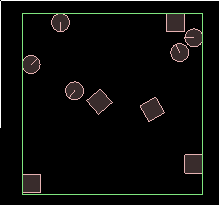Now we need a ray to cast against these shapes. Let's make a ray starting from the center of the screen and going outward, and rotating slowly around. The only state we need to keep for this is the current angle, so instead of making a special class for it, we'll just keep a variable at global scope.
 ```1 2 3 4 5 6 7 8 9 10 11 12 13 14 15 16 17 ``` ```  //at global scope   float currentRayAngle = 0;     //in Step() function   currentRayAngle += 360 / 20.0 / 60.0 * DEGTORAD; //one revolution every 20 seconds     //calculate points of ray   float rayLength = 25; //long enough to hit the walls   b2Vec2 p1( 0, 20 ); //center of scene   b2Vec2 p2 = p1 + rayLength * b2Vec2( sinf(currentRayAngle), cosf(currentRayAngle) );     //draw a line   glColor3f(1,1,1); //white   glBegin(GL_LINES);   glVertex2f( p1.x, p1.y );   glVertex2f( p2.x, p2.y );   glEnd();```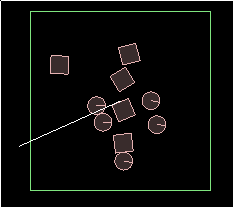You should now see a white line circling the scene. Now we just need to use the RayCast function to get the distance to the closest intersected shape, and draw the line at that length. We will check every fixture of every shape, which is not the best way to do this, but will do as an example (see the world querying topic for a more efficient method). It also means we can take a look at how you can iterate over the contents of the world:
 ```1 2 3 4 5 6 7 8 9 10 11 12 13 14 15 16 17 18 19 20 21 22 23 24 25 26 27 28 29 30 31 32 33 34 35 36 37 38 ``` ```  //in Step() function, continuing on from section above         //set up input       b2RayCastInput input;       input.p1 = p1;       input.p2 = p2;       input.maxFraction = 1;         //check every fixture of every body to find closest       float closestFraction = 1; //start with end of line as p2       b2Vec2 intersectionNormal(0,0);       for (b2Body* b = m_world->GetBodyList(); b; b = b->GetNext()) {           for (b2Fixture* f = b->GetFixtureList(); f; f = f->GetNext()) {                 b2RayCastOutput output;               if ( ! f->RayCast( &output, input ) )                   continue;               if ( output.fraction < closestFraction ) {                   closestFraction = output.fraction;                   intersectionNormal = output.normal;               }                       }       }         b2Vec2 intersectionPoint = p1 + closestFraction * (p2 - p1);         //draw a line       glColor3f(1,1,1); //white       glBegin(GL_LINES);       glVertex2f( p1.x, p1.y );       glVertex2f( intersectionPoint.x, intersectionPoint.y );       glEnd();             //draw a point at the intersection point       glPointSize(5);       glBegin(GL_POINTS);       glVertex2f( intersectionPoint.x, intersectionPoint.y );       glEnd();```
You might notice that now we are drawing two lines on top of each other... for clarity, delete the first one and you should get this result: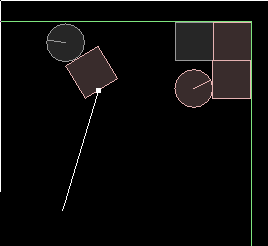Well that's about all there is to finding the intersection point. We can use the normal in the output struct for some interesting stuff though, so let's try it out. First, we'll simply render the normal to see what it looks like:
 ```1 2 3 4 5 6 7 ``` ```      //draw intersection normal       b2Vec2 normalEnd = intersectionPoint + intersectionNormal;       glColor3f(0,1,0); //green       glBegin(GL_LINES);       glVertex2f( intersectionPoint.x, intersectionPoint.y );       glVertex2f( normalEnd.x, normalEnd.y );       glEnd();```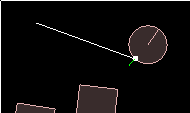And for a grand finale, we can put the raycasting code into its own function and call it recursively to reflect the ray off the intersected fixtures until it runs out. This is not really anything to do with Box2D, I just think it's neat :)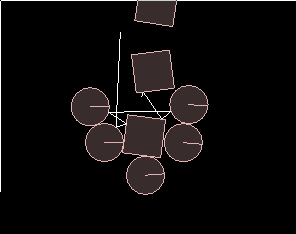Here is the code:
 ```1 2 3 4 5 6 7 8 9 10 11 12 13 14 15 16 17 18 19 20 21 22 23 24 25 26 27 28 29 30 31 32 33 34 35 36 37 38 39 40 41 42 43 44 45 ``` ```  //new function for FooTest class   void drawReflectedRay( b2Vec2 p1, b2Vec2 p2 )   {       //set up input       b2RayCastInput input;       input.p1 = p1;       input.p2 = p2;       input.maxFraction = 1;         //check every fixture of every body to find closest       float closestFraction = 1; //start with end of line as p2       b2Vec2 intersectionNormal(0,0);       for (b2Body* b = m_world->GetBodyList(); b; b = b->GetNext()) {           for (b2Fixture* f = b->GetFixtureList(); f; f = f->GetNext()) {                 b2RayCastOutput output;               if ( ! f->RayCast( &output, input ) )                   continue;               if ( output.fraction < closestFraction ) {                   closestFraction = output.fraction;                   intersectionNormal = output.normal;               }           }       }       b2Vec2 intersectionPoint = p1 + closestFraction * (p2 - p1);         //draw this part of the ray       glBegin(GL_LINES);       glVertex2f( p1.x, p1.y );       glVertex2f( intersectionPoint.x, intersectionPoint.y );       glEnd();         if ( closestFraction == 1 )           return; //ray hit nothing so we can finish here       if ( closestFraction == 0 )           return;         //still some ray left to reflect       b2Vec2 remainingRay = (p2 - intersectionPoint);       b2Vec2 projectedOntoNormal = b2Dot(remainingRay, intersectionNormal) * intersectionNormal;       b2Vec2 nextp2 = p2 - 2 * projectedOntoNormal;         //recurse       drawReflectedRay(intersectionPoint, nextp2);   }```
... and then inside Step() you would just have:
 ```1 2 3 4 5 6 7 ``` ```      //calculate points of ray       float rayLength = 25;       b2Vec2 p1( 0, 20 ); //center of scene       b2Vec2 p2 = p1 + rayLength * b2Vec2( sinf(currentRayAngle), cosf(currentRayAngle) );         glColor3f(1,1,1); //white       drawReflectedRay(p1, p2);```